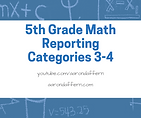5.4h

represent and solve problems related to perimeter and/or area and related to volume

5.8c; 5.9a

graph in the first quadrant of the coordinate plane ordered pairs of numbers arising from mathematical and real-world problems, including those generated by number patterns or found in an input-output table; represent categorical data with bar graphs or frequency tables and numerical data, including data sets of measurements in fractions or decimals, with dot plots or stem-and-leaf plots

5.5a; 5.6a

classify two-dimensional figures in a hierarchy of sets and subsets using graphic organizers based on their attributes and properties; recognize a cube with side length of one unit as a unit cube having one cubic unit of volume and the volume of a three-dimensional figure as the number of unit cubes (n cubic units) needed to fill it with no gaps or overlaps if possible

5.9b; 5.9c

represent discrete paired data on a scatterplot; solve one- and two-step problems using data from a frequency table, dot plot, bar graph, stem-and-leaf plot, or scatterplot

5.6b; 5.7a

determine the volume of a rectangular prism with whole number side lengths in problems related to the number of layers times the number of unit cubes in the area of the base; solve problems by calculating conversions within a measurement system, customary or metric

5.10a; 5.10b

define income tax, payroll tax, sales tax, and property tax; explain the difference between gross income and net income

5.8a; 5.8b

describe the key attributes of the coordinate plane, including perpendicular number lines (axes) where the intersection (origin) of the two lines coincides with zero on each number line and the given point (0, 0); the x- coordinate, the first number in an ordered pair, indicates movement parallel to the x- axis starting at the origin; and the y- coordinate, the second number, indicates movement parallel to the y- axis starting at the origin; describe the process for graphing ordered pairs of numbers in the first quadrant of the coordinate plane;

5.10e; 5.10f

describe actions that might be taken to balance a budget when expenses exceed income; balance a simple budget

Quizzes

Click the button below to access the quizzes for each part. Credit will not be earned until the CPE course has been purchased and all quizzes have been completed.

Take the 11 hour CPE course (\$36)

5th Grade Math Reporting Categories 3 - 4